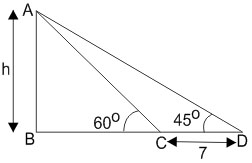#### AB is a vertical pole with  B at the ground level and A at the top. A man finds that the angle of elevation of the point ,A  from a certain point C   on the ground is. He moves away from the pole along the line BC  to the point D  such that  CD=7m. From D The angle of elevation of the point A   is 45°. Then the height of the pole is Option 1)Option 2)Option 3)Option 4)As we leant in

Height and Distances -

The height or length of an object or the distance between two distant objects can be determined with the help of trigonometric ratios.

-

Let height be hNow,Option 1)This option is incorrect

Option 2)This option is incorrect.

Option 3)This option is correct.

Option 4)This option is incorrect.

##### Posted by